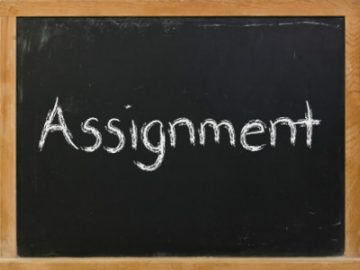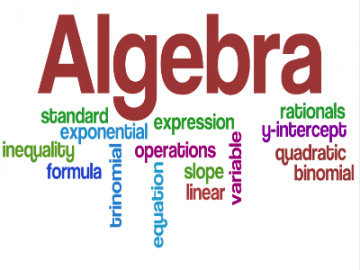## Basic Math Exercise

Basic Math Exercise Basic Math Exercise Mixed Practice Exercise: Simplify the following:   Answer Mixed Practice:   Answer Explanations:   Other basic math practice: Please solve the following without any calculator: Practice 1: Simplify the following expressions: 1. 39 – (25 – 17) 2. 3 (4 – 2) ÷ 2 Read More …

## Basic Math Lesson-01

Basic Math Lesson-01 Basic Quantitative Reasoning Welcome to Basic Math Lesson-01, which is the first of the three lessons that covers basics of Quantitative Reasoning section. The access to only first lesson is for FREE. These beginners lessons are very helpful for many tests that are required for admission in Read More …

## GAT Algebra Practice Question 2

GAT Algebra Practice Question 2 GAT Algebra Practice Question 2 Firstly, Today’s GAT algebra practice problem #2 is a problem solving question on word Problems. Try solving it first before you look at the explanation below. Question: Ahmed has exactly enough money to purchase meal for 6 people. If the Read More …

## GAT Quantitative Assignments-03## GAT Quantitative Assignments-02